# Introduction to R

### karthik.ram+R@gmail.com

M to see all slides, G to go to a specific slide

R is a language that's easy to learn badly

## Why R?

### The old way...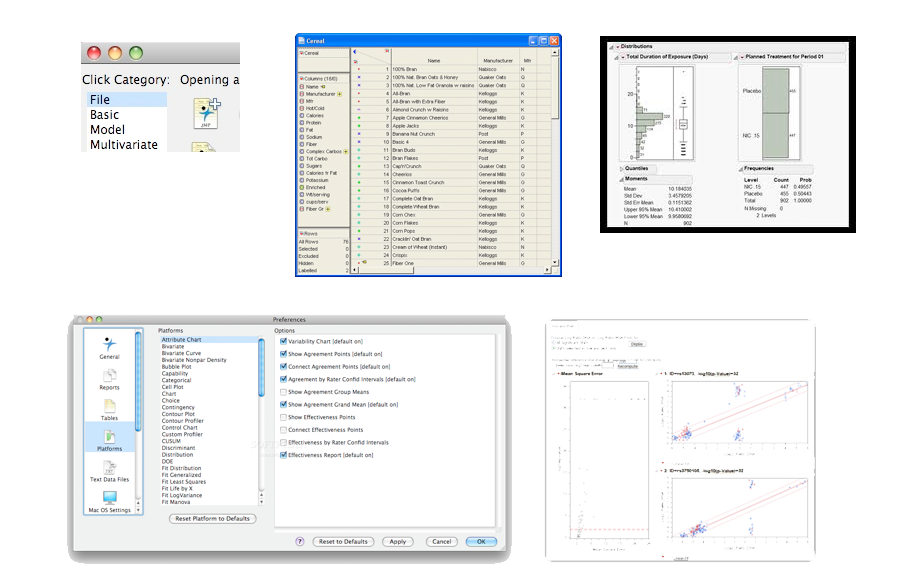## Why R?

### A better way

```glm(y ~ -1 + a + c + z + a:z, data = mydata, maxit = 30)
```

## Choose an appropriate code editor

1. ### R Studio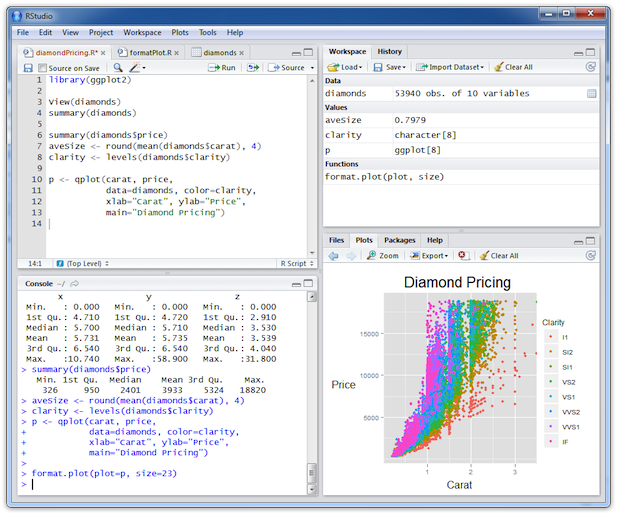1. ### TinnR (Windows), Sublime Text (all), Text Wrangler (osx) etc.

All come with key bindings. Pick one that you like.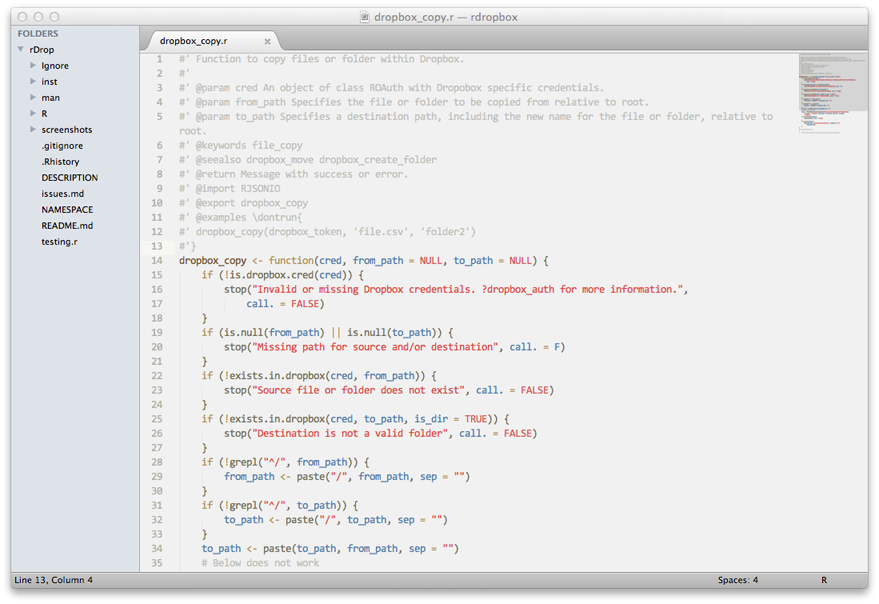## A sample workflow

 ```load.r load all raw untransformed data. ``` → ```clean.r Transform and clean messy data incl. missing values. ``` → ```functions.r Keep all functions in one separate file. ``` → ```do.r Your actual analysis. Load cleaned data and source all functions. ```

```# File: do.r
source("functions.r")
```

### Avoid restoring workspaces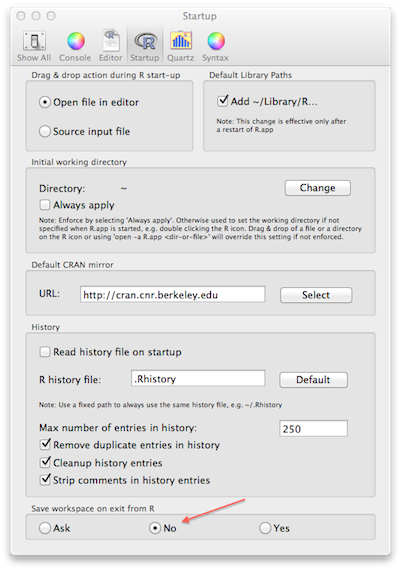## Workflow...

### Set working directores from the console and not scripts

```# My_script.r
setwd("path/to/dir")
# Removing any extra objects from my workspace (just in case)
rm(list = ls())
# Keeps my current file clutter free
source("related_r_scripts.r")
```

## Workflow...

### Never attach data

```attach(mydata)
```

### Always refer to it explicitly

```mydata\$column_name
```

## Environments and Namespaces

 ```Global Environment a <- rnorm(100) ``` ```Function or Namespace a <- 5 ```

 ```Good ✔# Script to analyze rainfall data. # loading lib: plyr_1.7.1, devtools_0.6, ggplot2_0.9.0 library(stringr) library(ggplot2) library(plyr) # reading previously cleaned data load("data_files.rdata") # Sourcing functions source("functions.r") # Setting up parameters annual_mean <- 25 # mean value is in cm ``` ```Bad ✖library(ggplot2) foo <- read.csv("file1.csv") a <- 1 b <- 3 test <- function(foo2) { return(a + b) } ```

## Quick guide to R data types

```# Vector: (single dimension, all same type)
vec1 <- 1:10
class(vec1)
##  "integer"
vec2 <- letters[1:10]
class(vec2)
##  "character"
# Data Frame: Each column is a vector, but adjacent vectors can hold different things
# Matrix: Just like a data.frame except it's all numeric
# List: (any dimension, mix and match)
l1 <- list(A = data.frame(x = 1:10, y = rnorm(10)),
B = 1, C = letters[1:3])
str(l1)
## List of 3
\$ A:'data.frame':	10 obs. of  2 variables:
..\$ x: int [1:10] 1 2 3 4 5 6 7 8 9 10
..\$ y: num [1:10] 0.618 0.519 0.343 0.428 -0.885 ...
\$ B: num 1
\$ C: chr [1:3] "a" "b" "c"
```

## Finding Help

### Locally

``````
?function_name
??function_name
RSiteSearch("function_name")
``````

### Online

 StackOverflow.com/questions/tagged/r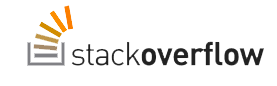Rseek.org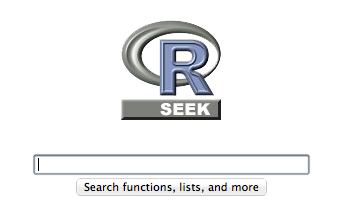## `sessionInfo()`

```sessionInfo()
## R version 2.14.2 (2012-02-29)
Platform: x86_64-apple-darwin9.8.0/x86_64 (64-bit)

locale:
 en_US.UTF-8/en_US.UTF-8/en_US.UTF-8/C/en_US.UTF-8/en_US.UTF-8

attached base packages:
 graphics  grDevices utils     datasets  stats     methods   base

other attached packages:
 knitr_0.3      formatR_0.3-4  devtools_0.6   plyr_1.7.1
 reshape2_1.2.1 ggplot2_0.9.0

loaded via a namespace (and not attached):
 codetools_0.2-8    colorspace_1.1-1   dichromat_1.2-4
 digest_0.5.1       evaluate_0.4.1     gdata_2.8.2
 grid_2.14.2        gtools_2.6.2       highlight_0.3.1
 MASS_7.3-17        memoise_0.1        munsell_0.3
 parser_0.0-14      proto_0.3-9.2      RColorBrewer_1.0-5
 Rcpp_0.9.10        RCurl_1.91-1       scales_0.2.0
 stringr_0.6        tools_2.14.2
```

## Use `dput()` to share some data

```dput(head(mtcars))
structure(list(mpg = c(21, 21, 22.8, 21.4, 18.7, 18.1), cyl = c(6,
6, 4, 6, 8, 6), disp = c(160, 160, 108, 258, 360, 225), hp = c(110,
110, 93, 110, 175, 105), drat = c(3.9, 3.9, 3.85, 3.08, 3.15,
2.76), wt = c(2.62, 2.875, 2.32, 3.215, 3.44, 3.46), qsec = c(16.46,
17.02, 18.61, 19.44, 17.02, 20.22), vs = c(0, 0, 1, 1, 0, 1),
am = c(1, 1, 1, 0, 0, 0), gear = c(4, 4, 4, 3, 3, 3), carb = c(4,
4, 1, 1, 2, 1)), .Names = c("mpg", "cyl", "disp", "hp", "drat",
"wt", "qsec", "vs", "am", "gear", "carb"), row.names = c("Mazda RX4",
"Mazda RX4 Wag", "Datsun 710", "Hornet 4 Drive", "Hornet Sportabout",
"Valiant"), class = "data.frame")
```

## Leverage your `.rprofile`

### Set `options`

```options(max.print = 2000)
options(prompt = "\$ ")
options(stringsAsFactors = FALSE)
# Store API keys
options(MendeleyKey = "My_secret_key")

```
``````
options() to list current settings
``````

## Leverage your `.rprofile`

```
library(ggplot2)
library(stringr)
library(plyr)
library(devtools)
```

## Leverage your `.rprofile`

```# A function that tells me which packages are out of date
check.packages <- function() {
if (!is.null(utils::old.packages())) {
old_packages <- utils::old.packages()
cat("Notification:", dim(old_packages), "packages are out of date \n")
cat(unname(old_packages[, 1]), sep = ",", "\n")
}
if (is.null(utils::old.packages())) {
cat("All packages are current \n")
}
}
```

## Word of caution regarding`.rprofile`

While the `.rprofile` does make life convenient, remember that any code/settings stored there are not reproducible by others.

```
# To load R without the .rprofile
`R -- vanilla`
```

Thanks to Hadley for pointing this out oversight.

### from flat text files

``read.table``

### from databases

``Use packages RODBC, RMySQL``

### from cloud storage

``Amazon S3, Google Docs, Dropbox etc.``

## Saving Data

Short-term
```save(data, file = "slots.rdata")
```
Long-term
```write.table(data, file = "slots-3.csv", sep = ",",
row = F)
```

## Manipulating Data - Plyr

The Split-Apply strategy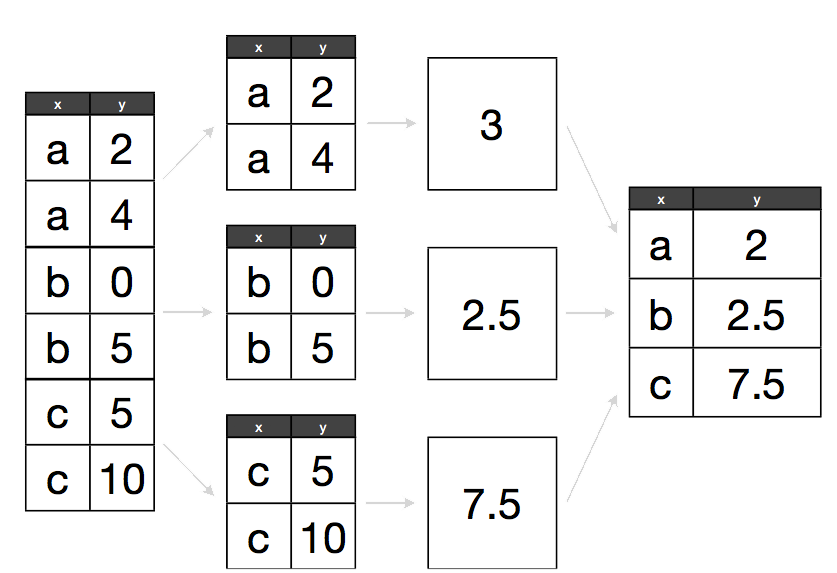## ddply example

```data <- data.frame(x = c("a", "a", "b", "b", "c",
"c"), y = c(2, 4, 0, 5, 5, 10))
```
```ddply(data, .(x), summarise, y = mean(y))
```

## Plyr syntax

Function naming scheme: first letter of source R object + first letter of output R object + ply

```result <- ddply(data, variable, summarise, n = sum(n))
```
```result <- llply(list_name, function_name)
```

## reshape2 allows you to reshape data into any format possible

```dcast(melted_data, temp ~ light, length)
```
```dcast(melted_data, temp ~ light, mean)
```
```dcast(melted_data, temp ~ light, custom_function)
```

## Example of melting and casting

```test_data <- data.frame(id = 1:9, category = factor(rep(sample(letters[1:3]),
3)), treatment = rep(sample(c("control", "trt_1", "trt_2")),
3), price_index = rnorm(9) * 200, prev_yr_index = rnorm(9) *
200)
```
```melted_data <- melt(test_data, id.vars = 1:3)
```
```dcast(melted_data, category + treatment ~ variable,
length)
dcast(melted_data, category + treatment ~ variable,
mean)
```

## Writing Functions

If you have to repeat the same 3-4 lines of code more than once, turn it into a function

## Use a version control system like git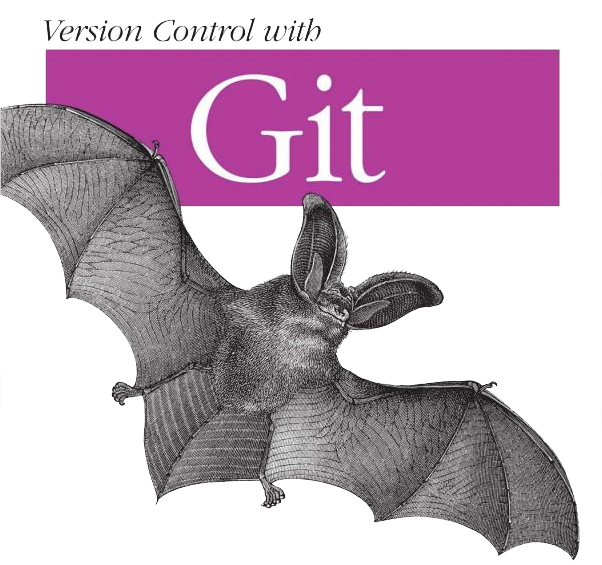## Dynamic report generation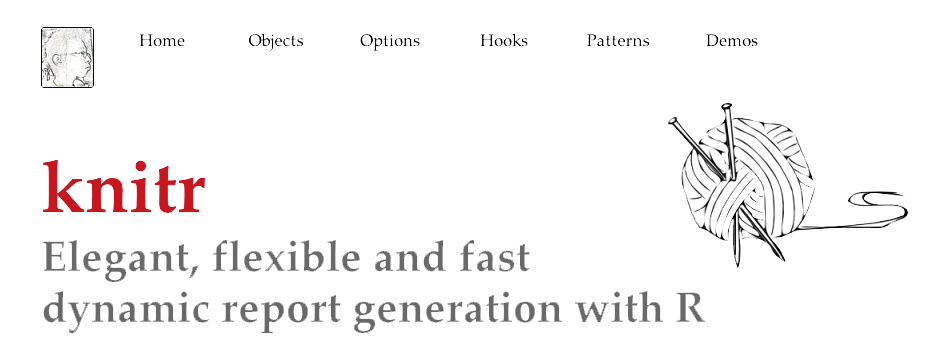``````
# As easy as:
knit('report.rnw')

# All the syntax in this talk was generated using knitr
``````

## R on the cloud

### R scales really well on cloud platforms (AWS, most anything)## Make function calls to R using REST API

### opencpu.org/

#